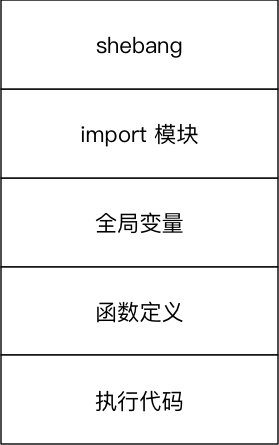• 累计撰写 270 篇文章
• 累计创建 6 个标签
• 累计收到 1 条评论

### 目 录CONTENT# Python中的高阶变量.md2021-08-22 / 0 评论 / 0 点赞 / 3 阅读 / 3,143 字 / 正在检测是否收录...

@[toc]

# 变量进阶

## 01. 变量的引用

• 变量 和 数据 都是保存在 内存 中的
• Python函数 的 参数传递 以及 返回值 都是靠 引用 传递的

### 1.1 引用的概念

Python

• 变量数据 是分开存储的
• 数据 保存在内存中的一个位置
• 变量 中保存着数据在内存中的地址
• 变量记录数据的地址，就叫做 引用
• 使用 id() 函数可以查看变量中保存数据所在的 内存地址

• 变量 不再 对之前的数据引用
• 变量 改为 对新赋值的数据引用

### 1.3 函数的参数和返回值的传递

Python 中，函数的 实参/返回值 都是是靠 引用 来传递来的

def test(num):

print("-" * 50)
print("%d 在函数内的内存地址是 %x" % (num, id(num)))

result = 100

print("返回值 %d 在内存中的地址是 %x" % (result, id(result)))
print("-" * 50)

return  result

a = 10
print("调用函数前 内存地址是 %x" % id(a))

r = test(a)

print("调用函数后 实参内存地址是 %x" % id(a))
print("调用函数后 返回值内存地址是 %x" % id(r))



## 02. 可变和不可变类型

• 不可变类型，内存中的数据不允许被修改：

• 数字类型 int, bool, float, complex, long(2.x)
• 字符串 str
• 元组 tuple
• 可变类型，内存中的数据可以被修改：

• 列表 list
• 字典 dict
a = 1
a = "hello"
a = [1, 2, 3]
a = [3, 2, 1]

demo_list = [1, 2, 3]

print("定义列表后的内存地址 %d" % id(demo_list))

demo_list.append(999)
demo_list.pop(0)
demo_list.remove(2)
demo_list = 10

print("修改数据后的内存地址 %d" % id(demo_list))

demo_dict = {"name": "小明"}

print("定义字典后的内存地址 %d" % id(demo_dict))

demo_dict["age"] = 18
demo_dict.pop("name")
demo_dict["name"] = "老王"

print("修改数据后的内存地址 %d" % id(demo_dict))



1. 可变类型的数据变化，是通过 方法 来实现的
2. 如果给一个可变类型的变量，赋值了一个新的数据，引用会修改
• 变量 不再 对之前的数据引用
• 变量 改为 对新赋值的数据引用

### 哈希 (hash)

• Python 中内置有一个名字叫做 hash(o) 的函数
• 接收一个 不可变类型 的数据作为 参数
• 返回 结果是一个 整数
• 哈希 是一种 算法，其作用就是提取数据的 特征码（指纹）
• 相同的内容 得到 相同的结果
• 不同的内容 得到 不同的结果
• Python 中，设置字典的 键值对 时，会首先对 key 进行 hash 已决定如何在内存中保存字典的数据，以方便 后续 对字典的操作：增、删、改、查
• 键值对的 key 必须是不可变类型数据
• 键值对的 value 可以是任意类型的数据

## 03. 局部变量和全局变量

• 局部变量 是在 函数内部 定义的变量，只能在函数内部使用
• 全局变量 是在 函数外部定义 的变量（没有定义在某一个函数内），所有函数 内部 都可以使用这个变量

### 3.1 局部变量

• 局部变量 是在 函数内部 定义的变量，只能在函数内部使用
• 函数执行结束后，函数内部的局部变量，会被系统回收
• 不同的函数，可以定义相同的名字的局部变量，但是 彼此之间 不会产生影响

#### 局部变量的作用

• 在函数内部使用，临时 保存 函数内部需要使用的数据
def demo1():

num = 10

print(num)

num = 20

print("修改后 %d" % num)

def demo2():

num = 100

print(num)

demo1()
demo2()

print("over")



#### 局部变量的生命周期

• 所谓 生命周期 就是变量从 被创建被系统回收 的过程
• 局部变量函数执行时 才会被创建
• 函数执行结束后 局部变量 被系统回收
• 局部变量在生命周期 内，可以用来存储 函数内部临时使用到的数据

### 3.2 全局变量

• 全局变量 是在 函数外部定义 的变量，所有函数内部都可以使用这个变量
# 定义一个全局变量
num = 10

def demo1():

print(num)

def demo2():

print(num)

demo1()
demo2()

print("over")



1. 首先 查找 函数内部 是否存在 指定名称 的局部变量如果有，直接使用
2. 如果没有，查找 函数外部 是否存在 指定名称 的全局变量如果有，直接使用
3. 如果还没有，程序报错！

#### 1) 函数不能直接修改 全局变量的引用

• 全局变量 是在 函数外部定义 的变量（没有定义在某一个函数内），所有函数 内部 都可以使用这个变量

• 在函数内部，可以 通过全局变量的引用获取对应的数据
• 但是，不允许直接修改全局变量的引用 —— 使用赋值语句修改全局变量的值
num = 10

def demo1():

print("demo1" + "-" * 50)

# 只是定义了一个局部变量，不会修改到全局变量，只是变量名相同而已
num = 100
print(num)

def demo2():

print("demo2" + "-" * 50)
print(num)

demo1()
demo2()

print("over")



#### 2) 在函数内部修改全局变量的值

• 如果在函数中需要修改全局变量，需要使用 global 进行声明
num = 10

def demo1():

print("demo1" + "-" * 50)

# global 关键字，告诉 Python 解释器 num 是一个全局变量
global num
# 只是定义了一个局部变量，不会修改到全局变量，只是变量名相同而已
num = 100
print(num)

def demo2():

print("demo2" + "-" * 50)
print(num)

demo1()
demo2()

print("over")



#### 3) 全局变量定义的位置

• 为了保证所有的函数都能够正确使用到全局变量，应该 将全局变量定义在其他函数的上方
a = 10

def demo():
print("%d" % a)
print("%d" % b)
print("%d" % c)

b = 20
demo()
c = 30



• 由于全局变量 c，是在调用函数之后，才定义的，在执行函数时，变量还没有定义，所以程序会报错！#### 4) 全局变量命名的建议

• 为了避免局部变量和全局变量出现混淆，在定义全局变量时，有些公司会有一些开发要求，例如：
• 全局变量名前应该增加 g_ 或者 gl_ 的前缀

0